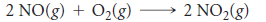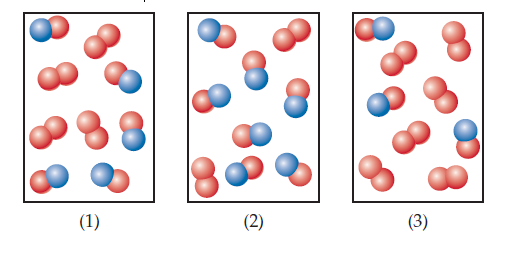×
Get Full Access to Chemistry: The Central Science - 12 Edition - Chapter 14 - Problem 6e
Get Full Access to Chemistry: The Central Science - 12 Edition - Chapter 14 - Problem 6e

×

# Visualizing ConceptsThe following diagrams representISBN: 9780321696724 27

## Solution for problem 6E Chapter 14

Chemistry: The Central Science | 12th Edition

• Textbook Solutions
• 2901 Step-by-step solutions solved by professors and subject experts
• Get 24/7 help from StudySoup virtual teaching assistantsChemistry: The Central Science | 12th Edition

4 5 1 253 Reviews
20
0
Problem 6E

The following diagrams represent mixtures of NO(g) and O2(g). These two substances react as follows:It has been determined experimentally that the rate is second order in NO and first order in O2. Based on this fact, which of the following mixtures will have the fastest initial rate? [Section 14.3]Step-by-Step Solution:
Step 1 of 3

MATH 2450 WEEK 6 Chain Rule in Higher Dimensions g(t) = df(x(t) , y(t))= ∂f * dx + ∂f * dy dt ∂x dt ∂y dt R(t) = < x(t), y(t), f( x(t), y(t) ) df(g(t)) = df * dg dt dg dt 2 EX. Use the chain rule to find the df/dt of the function f(x,y) = x + 3xy where x(t) = cos(t) and y(t) = sin(t) Fx= 2x + 3y Fx= 3x dx/dt = -sin(t) dy/dt = cos(t) Now plug in the values you found above into the chain rule equation g(t) = ∂f * dx + ∂f * dy ∂x dt ∂y dt g(t) = (2x + 3y) (-sin(t)) + (3x) (cos(t)) Now make everything is terms of ‘t’. Remember to replace the ‘x’ and ‘y’ with their ORIGINAL values(x(t) = cos(t) and y(t) = sin(t)) not their derivatives g(t) = (2cos(t) + 3sin(t)) (-sin(t)) + (3cos(t)) (cos(t)) g(t) = -2sin(t)cos(t) – 3sin (t) + 3cos (t) Chain Rule in higher dimensions with 3 variables F(x,y,z)  R(t) = (t) = df/dt = x * x’(t) +yf * y’(t) z f * z’(t) EX. Use the chain rule to find the df/dt of the function f(x, y, z) = e x*y^(2)*zwhere x(t)= t ,2 y(t)=e, and z(t) = cos(t) 2 x*y^(2)*z F x y *z*e F y 2*x*y*e x*y^(2)*z F z y *x*e x*y^(2)*z x’(t) = 2t y’(t) = e t z’(t) = -sin(t) Plug in the values into the chain rule equation df/dt = f x x’(t) + f *yy’(t) + f * zz(t) = (y *z*e x*y^(2)*)(2t) + (2*x*y*e x*y^(2)*)(e) + (y *x*e x*y^(2)*)(-sin(t))

Step 2 of 3

Step 3 of 3

##### ISBN: 9780321696724

The answer to “?The following diagrams represent mixtures of NO(g) and O2(g). These two substances react as follows: It has been determined experimentally that the rate is second order in NO and first order in O2. Based on this fact, which of the following mixtures will have the fastest initial rate? [Section 14.3]” is broken down into a number of easy to follow steps, and 50 words. This full solution covers the following key subjects: Mixtures, rate, order, initial, Diagrams. This expansive textbook survival guide covers 49 chapters, and 5471 solutions. The full step-by-step solution to problem: 6E from chapter: 14 was answered by , our top Chemistry solution expert on 04/03/17, 07:58AM. Since the solution to 6E from 14 chapter was answered, more than 464 students have viewed the full step-by-step answer. Chemistry: The Central Science was written by and is associated to the ISBN: 9780321696724. This textbook survival guide was created for the textbook: Chemistry: The Central Science, edition: 12.

Unlock Textbook Solution